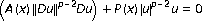# Riccati inequality and oscillation criteria for PDE with-Laplacian

## Abstract

Oscillation criteria for PDE with-Laplacian divare obtained via Riccati inequality. Some of them are extensions of the results for the second-order linear ODE to this equation.



## References

1. Díaz JI: Nonlinear Partial Differential Equations and Free Boundaries. Vol. I. Elliptic Equations, Research Notes in Mathematics. Volume 106. Pitman (Advanced Publishing Program), Massachusetts; 1985:vii+323.

2. Kong Q: Interval criteria for oscillation of second-order linear ordinary differential equations. Journal of Mathematical Analysis and Applications 1999,229(1):258–270. 10.1006/jmaa.1998.6159

3. Philos ChG: Oscillation theorems for linear differential equations of second order. Archiv der Mathematik. Archives of Mathematics. Archives Mathématiques 1989,53(5):482–492.

4. Usami H: Some oscillation theorems for a class of quasilinear elliptic equations. Annali di Matematica Pura ed Applicata. Series IV 1998, 175: 277–283. 10.1007/BF01783687

5. Wintner A: A criterion of oscillatory stability. Quarterly of Applied Mathematics 1949, 7: 115–117.

6. Wong JSW: On Kamenev-type oscillation theorems for second-order differential equations with damping. Journal of Mathematical Analysis and Applications 2001,258(1):244–257. 10.1006/jmaa.2000.7376

7. Xu Z-T, Xing H-Y: Oscillation criteria of Kamenev-type for PDE with-Laplacian. Applied Mathematics and Computation 2003,145(2–3):735–745. 10.1016/S0096-3003(03)00270-4

## Author information

Authors

### Corresponding author

Correspondence to Zhiting Xu.

## Rights and permissions

Reprints and Permissions

Xu, Z. Riccati inequality and oscillation criteria for PDE with-Laplacian. J Inequal Appl 2006, 63061 (2006). https://doi.org/10.1155/JIA/2006/63061

• Revised:

• Accepted:

• Published:

• DOI: https://doi.org/10.1155/JIA/2006/63061

### Keywords

• Oscillation Criterion
• Riccati Inequality